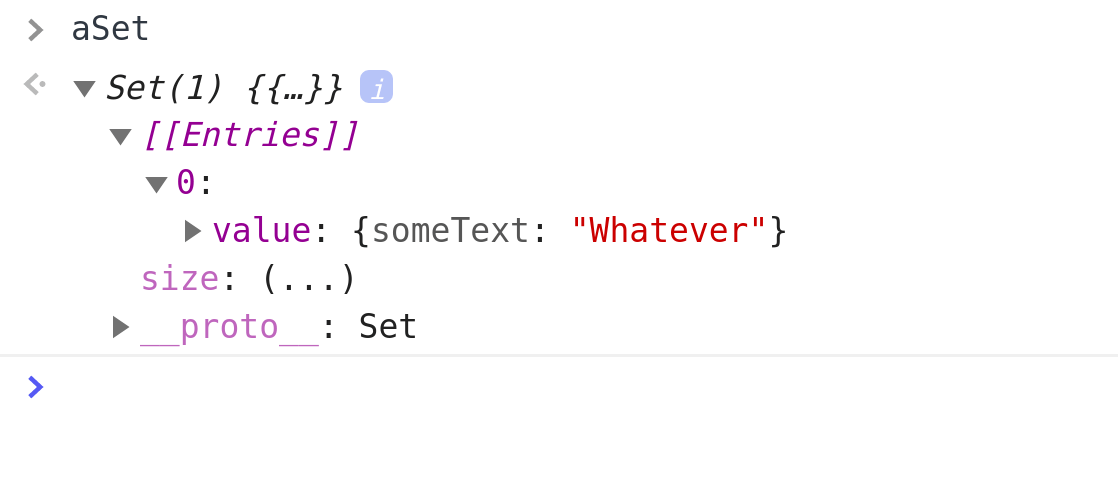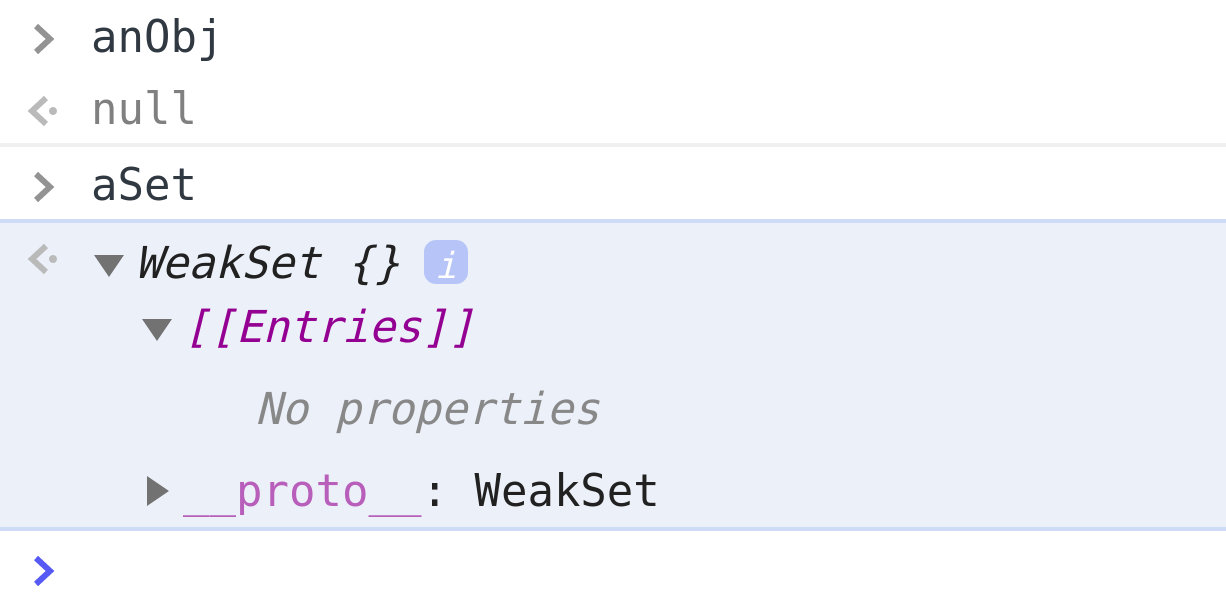In this article, we’ll have a look at quite a few JavaScript quirks.Image by CodingExercises

## A Set is a Collection of Unique Members

To create a set, we use the `Set()` constructor.

``````let daysOfTheWeek = new Set();
``````

To populate a set, we use the `add` method.

``````daysOfTheWeek.add('Mon');
daysOfTheWeek; // returns: Set(1) { 'Mon' }
``````

A useful thing about sets is that if you already have a value inside of a set, using the `add` method to add an exact same value is not possible; the JavaScript engine will simply ignore it.

For example, if we continue the above code by adding Tuesday, it will work:

``````daysOfTheWeek.add('Tue');
daysOfTheWeek; // returns: Set(2) {"Mon", "Tue" }
``````

However, re-adding Monday to our set will simply be ignored:

``````daysOfTheWeek.add('Mon');
daysOfTheWeek; // still returns: Set(2) {"Mon", "Tue" }
``````

## Convert a set to an array

To convert a set to an array, we can:

• use the Array.from method

With the spread operator, it’s simple:

``````let a = [...daysOfTheWeek];
a; // returns an array of values from the original set in the form of an array
``````

It’s not much harder using the Array.from method either:

``````let arr = Array.from(daysOfTheWeek);
arr; // returns an array of values from the original set in the form of an array
``````

## Convert an array to a set

To convert an array to a set, we can just pass the array to a set constructor:

``````let arr = ['one', 'two', 'three', 'one', 'two', 'three'];
let set = new Set(arr);
set; // returns a Set with values same as in the array
``````

## Remove duplicates from an array using a set

Now for a fun part. We can easily remove duplicates from an array by converting it to a set, then back to array.

Here it is:

``````let arr = ['one', 'two', 'three', 'one', 'two', 'three'];
let set = new Set(arr);
let arr2 = [...set];
arr; // returns: the original array with 6 members
arr2; // returns: a filtered array with 3 unique members
``````

## Just for fun (re-duplicate an array)

Let’s try to do the opposite, and re-duplicate array members, with a twist: we’ll do that only if they’re numbers, otherwise we’ll remove them.

Here’s one approach:

``````let arr = [1,2,3, 'a'];
arr = arr.join('');
arr = arr.repeat(2);
arr = [...arr]; // returns: ["1", "2", "3", "a", "1", "2", "3", "a"]
arr = arr.map(x => +x); // returns: [1,2,3,NaN,1,2,3,NaN]
arr = arr.filter(x => !Number.isNaN(x)); // returns: [1,2,3,1,2,3]
``````

## Does a value exist in a set?

Let’s add a new array, we’ll call it `arr`. We’ll also add a new set and call it `set`.

``````let arr = ['Mon', 'Tue', 'Wed'];
let set = new Set();
``````

Now we can check if the set has a `'Mon'` string.

``````set.has('Mon');
``````

We can also check if an array includes a `'Mon'`.

``````arr.includes('Mon');
``````

Here’s a quick check to see if both of them have the `'Mon'` entry:

``````set.has('Mon') === arr.includes('Mon')
``````

## Sets don’t consider objects the same even if they hold the same values

Expectedly, all objects are unique, even if their values are exactly the same.

For example:

``````let set = new Set().add(['a','b','c']).add(['a','b','c']);
set; // returns Set { [ 'a', 'b', 'c', ], [ 'a', 'b', 'c' ]}
``````

## Check the length of a set

To check the length of a set, we use the `size` property. For example:

``````let arr = [1,2,3,4,5];
let set = new Set(arr);
set.size; // returns: 5
``````

## Using two sets to find the items that exist in both

Let’s say our first set has these values:

``````let arr1 = [1,2,3,9];
let set1 = new Set(arr1);
``````

Let’s say our second set has these values:

``````let arr2 = [4,5,6,9];
let set2 = new Set(arr2);
``````

We can now find the duplicate members of each set by doing this:

``````let duplicates = new Set( [...set1].filter( x => set2.has(x) ) );
console.log(duplicates);
``````

## Deleting set members

To delete items in a set, we, use `delete`, like this:

``````let set1 = ['Jan', 'Feb', 'Mar', 'Apr', 'May', 'May'];
set1.delete('Jan'); // returns: true
set1; // returns: {"Feb", "Mar", "Apr", "May"}
``````

Whenever a deletion was successful, the `delete` method returns `true`; otherwise, if the deletion was not successful, the `delete` method returns `false`.

Thus, you could quickly check if an array doesn’t contain a certain value, like this:

``````let set1 = new Set(['a','b','c','d']);
if (set1.delete('e') == false) {
console.log("The letter 'e' could not be found in the set");
}
``````

## Comparing sets and weak sets

Let’s discuss this piece of code:

``````let anObj = { someText: "Whatever" };

console.log(anObj); // returns: { someText: "Whatever" }

anObj = null; // reassign anObj to null

console.log(anObj); // returns: null
``````

Now, there is one important behavior in JavaScript to understand:

If we declare a value as a separate variable, then add that variable to a collection, normally, that new member of a collection will be reachable to us, even if the original value is deleted.

For example:

``````let anObj = { someText: "Whatever" }

let anArr = [ anObj ];

anObj = null; // reassign anObj to null

console.log(anObj); // returns null

// BUT:
console.log(anArr); // returns { someText: whatever }
``````

So, what we can conclude from the above code is that arrays hold onto their member objects strongly - meaning, the member objects are not garbage collected for as long as their containing collection exists (this collection being the `anArr` array in the example above). Simply put, the array members “live on” even after the original object binding they were added from is deleted (in the example above, the `anObj`).

Ok, that’s all good, but where do weak sets fit in here?

Contrary to sets, weak sets can only contain objects.

So why are they weak? Because weak sets hold onto their member objects weakly. Meaning, they allow their members to be garbage collected when the “original” object binding is deleted.

First, let’s see the same example as the one above, only this time with a regular set:

``````let anObj = { someText: "Whatever" }; // returns: undefined

let aSet = new Set(); // returns: undefined

// Let's add an object to the set:

// Let's reassign the original object binding:
anObj = null; // returns: null
``````

Now let’s check if the object still “lives on” in the set:

``````aSet; // returns: Set(1)
``````

Indeed, it does! If we twirl open the Set to inspect it, we’ll see:Image by CodingExercises

That’s why we can say that a set holds on to its members strongly.

Now let’s do the above example again, only this time, using a weak set. What do you expect will happen?

``````let anObj = { someText: "Whatever" }; // returns: undefined

let aSet = new WeakSet(); // returns: undefined

// Let's add an object to the set:

// Let's reassign the original object binding:
anObj = null; // returns: null
``````

Now let’s check if the object still “lives on” in the set:

``````aSet; // returns: WeakSet {}
``````

If we twirl open the returned `WeakSet`, here’s what we’ll see:Image by CodingExercises

Obviously, the weak set indeed lets objects be garbage collected and doesn’t help them “live on” after their source object binding was reassigned.

Why is this important? How can we use it?

## Practical uses of Weak Sets in JavaScript

There are many different uses for Weak Sets, but these concepts are a bit more advanced, so we’ll cover them in a different article.

You can find some use cases in this StackOverflow question.

## Looping over sets and weak sets

To loop over sets, use the `for of` or `forEach`.

Here’s an example with `for of`:

``````let arr = [1,2,3];
let numSet = new Set(arr);
for ( num of numSet ) { console.log( num ) };
``````

The above returns:

``````1
2
3
``````

Sets are enumerable, and that’s why it’s possible to loop over them like this.

We can also loop over sets using the `forEach` method:

``````let arr = [4,5,6];
let numSet = new Set(arr);
numSet.forEach( item => console.log(item) );
``````

The above code returns:

``````4
5
6
``````

Currently (beginning of 2020), there’s no way to loop over weak sets in JavaScript.